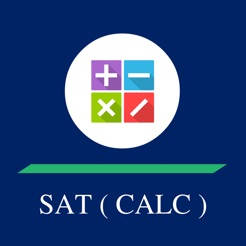## Screenshots

•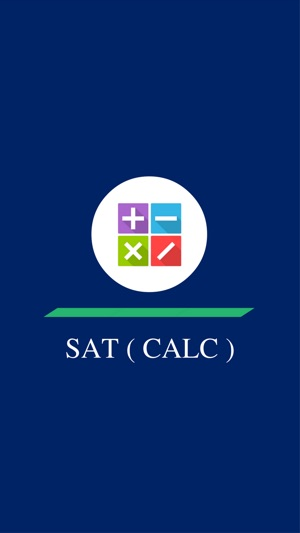•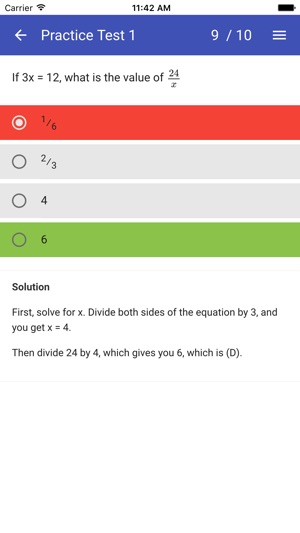•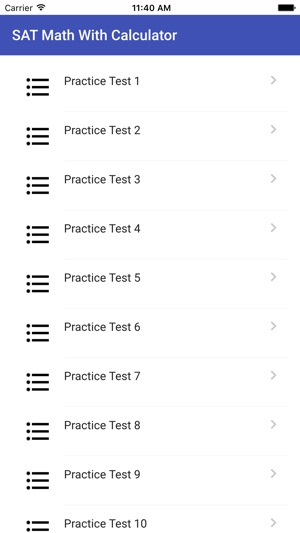•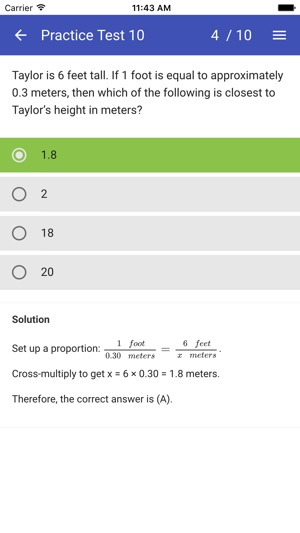•## Description

SAT Maths Practice Tests with Calculator app provide step by step solved practice papers using calculator. It's very useful to provide detailed guide for sat maths section. In Sat maths app, we walk through solving methods of practice problems covering all of the skills you’ll need for the SAT Math sections.

SAT Maths Calculator Sections Categories :-

1] Heart of Algebra :-

linear equations and inequalities, Interpreting linear functions, Linear equation word problems, Graphs based linear equations, Linear function problems, Systems of linear inequalities word problems, Solving methods of linear equations

2] Problem Solving and Data Analysis

Percents,Ratios, rates, and proportions, Units, Table data analysis, Scatterplots, graphs, Linear and exponential growth, Data inferences, Data collection & conclusions

3] Passport to Advanced Math

Solving quadratic equations, Interpreting nonlinear expressions, Quadratic and exponential word problems, Manipulating quadratic and exponential expressions, Radicals and rational exponents, Radical and rational equations, Operations with rational expressions, Operations with polynomials, Polynomial factors and graphs, Nonlinear equation graphs, Linear and quadratic systems, Structure in expressions, Isolating quantities, Function notation

4] Additional topics in Maths

Volume word problems, Right triangle word problems, Congruence and similarity, Right triangle trigonometry,Angles, Arc lengths, Trig functions, Circle equations, Complex numbers

## Information

Seller
devang patel
Size
12.3 MB
Category
Education
Compatibility

Requires iOS 9.0 or later. Compatible with iPhone, iPad, and iPod touch.

Languages

English

Age Rating
Rated 4+
Copyright
© Devang Patel
Price
Free

## Supports

•